# Lagrange bracket

Lagrange brackets, with respect to variablesandA sum of the form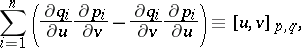(*)

where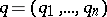andare certain functions ofand.

Ifand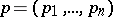are canonical variables and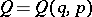,are canonical transformations, then the Lagrange bracket is an invariant of this transformation:For this reason the indiceson the right-hand side of (*) are often omitted. The Lagrange bracket is said to be fundamental when the variablesandcoincide with some pair of thevariables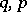. From them one can form three matrices: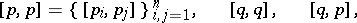the first two of which are the zero, and the last one is the unit matrix. There is a definite connection between Lagrange brackets and Poisson brackets. Namely, if the functions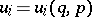,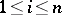, induce a diffeomorphism, then the matrices formed from the elements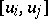andare inverse to each other.